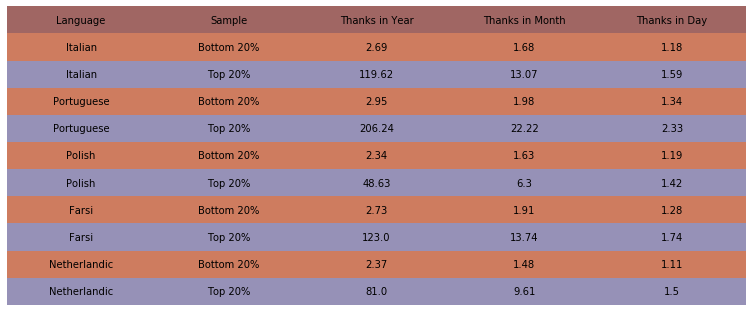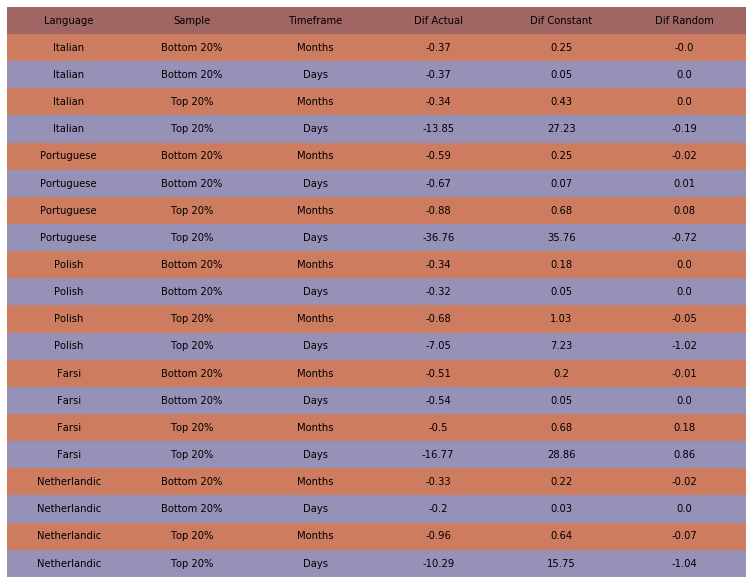#### Introduction¶

This study explores the distribution of thanks. The first figure presents data on the number of thanks the average person receives. The second figure presents data on whether thanks are received evenly throughout the year or in clusters.

#### SQL Query¶

• Gets all thanks in a timeframe

use PROJECT;

select B.user_editcount as Edit_Count, A.log_title as User_Name, A.log_timestamp as Thank_Time

from (select log_title, log_timestamp from logging_userindex where (log_action = 'thank' and log_type='thanks' and log_timestamp < timestamp(TIME1) and log_timestamp >= timestamp(TIME2))) as A

join (select user_editcount, user_name from user) as B

on A.log_title = B.user_name order by B.user_editcount, A.log_title

-- For this analysis: TIME1 = '2018-06-01' TIME2 = '2017-06-01' PROJECT = itwiki_p, ptwiki_p, plwiki_p, fawiki_p, nlwiki_p

import csv
from datetime import datetime
import random

#define filenames
src = '(1-4)-data/'
input_prefixes = ['It', 'Pt', 'Pl', 'Fa', 'Nl']
input_suffix = '.csv'

def make_files_lst(src=src, prefixes=input_prefixes, stem=input_stem, suffix=input_suffix):
lst = []
for prefix in prefixes:
lst.append(src+prefix+stem+suffix)
return lst

input_files = make_files_lst()

#information for breaking the data into percentiles
num_groups = 10
samples = [[0, 0.2], [0.8, 1]]
file_size, group_size, remainder = -1, -1, -1

def find_group_size(input_file, n):
#computes the values of:
global file_size, group_size, remainder
file_size = 0
with open(input_file, 'rt', encoding = 'utf-8') as csvfile:
for row in rder:
file_size += 1
group_size = int(file_size/n)
remainder = file_size - group_size * n

#calls spatial_distribution_inner() for all samples of all input files
def examine_spatial_distribution(input_files=input_files, samples=samples, num_groups=num_groups, simple=False):
data = []
for input_file in input_files:
for sample in samples:
start_group = round(sample * num_groups)
end_group = round(sample * num_groups)
d = spatial_distribution_inner(input_file, sample, start_group, end_group, simple)
data.append(d)
return data

#computes different statistics on spatial distribution (function has two modes: simple=True/False)
def spatial_distribution_inner(input_file, sample, start_group, end_group, simple):
with open(input_file, 'r', encoding = 'utf-8') as csvfile:
global file_size, group_size, remainder
find_group_size(input_file, num_groups)

i, j = 0, 0

thanks=0
people=0
months_by_person = {}
days_by_person = {}
thanks_by_person = {}

for row in rder:
i += 1
if (j >= start_group and j < end_group):
#set-up data collection for new user
people += 1
thanks += 1

#if this user has not yet received a thank on this month or day, increment the count
tme = row['Thank_Time'][:6]
if (not tme in months_by_person[username]):
tme = row['Thank_Time'][:8]
if (not tme in days_by_person[username]):

if (i == adjusted_group_size):
i = 0
j += 1
if (j == num_groups - remainder):

if (not simple):
#more complex mode, return differences in distribution with randomized and controlled data sets
return [find_average_difference(months_by_person, thanks_by_person, 12), find_average_difference(days_by_person, thanks_by_person, 365)]
else:
#simple mode, return average number of thanks/person for different timeframes
num_months, num_days = 0, 0
num_months = sum([len(months_by_person[k]) for k in months_by_person])*1.0/people
num_days = sum([len(days_by_person[k]) for k in days_by_person])*1.0/people
avg_thanks = thanks*1.0/people

return [avg_thanks, avg_thanks/num_months, avg_thanks/num_days]

#find average difference between the actual distribution and a random distribution,
#a random distribution and a random distribution, and a controlled distribution and a random distributioon
def find_average_difference(timespans_by_person, thanks_by_person, num_spans):
dif = 0
expected_dif = 0
normalized_dif = 0
usernames = [k for k in timespans_by_person]
random_timespans_by_person = generate_random_data_for_population(usernames, thanks_by_person, num_spans)
normalized_timespans_by_person = generate_normalized_data_for_population(usernames, thanks_by_person, num_spans)

random_spans = generate_random_data_for_person(thanks_by_person[username], num_spans)
dif += len(timespans_by_person[username]) - random_spans #actual - random
expected_dif += random_timespans_by_person[username] - random_spans #random - random
normalized_dif += normalized_timespans_by_person[username] - random_spans #controlled spread - random

#generate random data for the timeframes (months or days) in which a single person received thanks
def generate_random_data_for_person(num_thanks, num_spans):
random_spans = set()
for i in range(0, num_thanks):
return len(random_spans)

#generate random data for the timeframes (months or days) in which every person received thanks
def generate_random_data_for_population(usernames, thanks_by_person, num_spans):
spans = {}
return spans

#generate controlled (maximum-spread) data for the timeframes (months or days) in which every person received thanks
def generate_normalized_data_for_population(usernames, thanks_by_person, num_spans):
spans = {}
return spans

data = examine_spatial_distribution()

#define table inputs
columns = ['Language', 'Sample', 'Timeframe', 'Dif Actual', 'Dif Constant', 'Dif Random']
title = 'thanks-timeframe' #filename where table will be saved
languages = ['Italian', 'Portuguese', 'Polish', 'Farsi', 'Netherlandic']

#format data for table (round numbers, convert some numbers to labels)
def make_table(input_files=input_files, samples=samples, data=data, simple=False):
reformatted_data = []
for i in range(0, len(languages)):
for j in range(0, len(samples)):
if (j % 2 == 0):
sample = 'Bottom 20%'
else:
sample = 'Top 20%'
reformatted_data.append([languages[i], sample])
if (not simple):
reformatted_data.append([languages[i], sample])
for i in range(0, len(data)):
for j in range(0, len(data[i])):
if (not simple):
k = i*2+j
if (j % 2 == 0):
timeframe='Months'
else:
timeframe='Days'
reformatted_data[k].append(timeframe)
reformatted_data[k] += data[i][j]
else:
k = i
reformatted_data[k].append(data[i][j])
return reformatted_data

data = make_table()

import numpy as np
import pandas as pd
import matplotlib.pyplot as plt

#use reformatted data to create a table
def show_table(data=data, columns=columns, title=title, simple=False):
fig, ax = plt.subplots()

#hide axes
ax.axis('off')
ax.axis('tight')

#styling -- color cells by row, round all floats
colors = [['#9691b7']*len(data)]*len(data)
for i in range(0, len(colors)):
if (i % 2) == 0:
colors[i] = ['#ce7c5f']*len(data)
t = 3
if (simple):
t = 2
for i in range(0, len(data)):
for j in range(t, len(data[i])):
data[i][j] = round(data[i][j], 2)

df = pd.DataFrame(data, columns=columns)

table = ax.table(bbox=None, cellText=df.values, cellColours=colors, colColours=['#a06663']*len(columns), colLabels=df.columns, loc='center', cellLoc='center')

#styling -- get rid of lines in table
d = table.get_celld()
for k in d:
d[k].set_linewidth(0)

fig.tight_layout()
table.scale(2, 2)

plt.savefig('../figures/'+title+'.png', bbox_inches='tight')
plt.show()

simple = True

avgs_data = examine_spatial_distribution(simple=simple)

avgs_data = make_table(data=avgs_data, simple=simple)

#define table inputs
columns = ['Language', 'Sample', 'Thanks in Year', 'Thanks in Month', 'Thanks in Day']
title = 'thanks-avgs' #filename where table will be stored


Note: Only people who received a thank are represented in the data.

#figure 1, simpler analysis
show_table(avgs_data, columns, title, simple=simple)Note: The "Thanks in Month" and "Thanks in Day" numbers are averages by person only counting the months or days in which a person actually received a thank.

#figure 2, more complicated analysis
show_table()Note: Dif Actual is the difference between the average number of months (or days) on which people actually received thanks and the average number of months (or days) on which they would have received thanks if we spread thanks out randomly. Dif Constant is the difference between the average if we spread thanks out as much as possible and the average if we spread thanks out randomly. Dif Random is the difference between two random spreads.

##### Conclusion¶

Thanks appear to be more clustered than they would be if spread out over random days. In other words, people tend to receive thanks in clusters. Further analysis is needed to know exactly what these clusters look like.